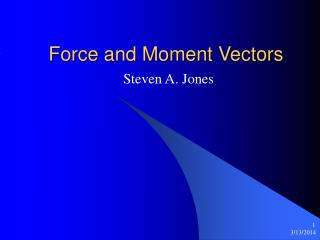DownloadDownload PresentationForce and Moment Vectors

# Force and Moment Vectors

Télécharger la présentation## Force and Moment Vectors

- - - - - - - - - - - - - - - - - - - - - - - - - - - E N D - - - - - - - - - - - - - - - - - - - - - - - - - - -
##### Presentation Transcript

1. Force and Moment Vectors Steven A. Jones

2. Basics • Force is rate of change of momentum • If you push on a wall, it exerts an equal force on you • Force is in Kg m/s2 or Newtons • Can also be g cm/s2 or dynes • A Newton is a little less than ¼ lbs, or a little less than the weight of ½ cup of water. • A dyne is 5 orders of magnitude smaller.

3. Forces • Forces add as vector quantities • Can draw a free body diagram • All forces must balance one another • Some forces may be discrete, some distributed. • With distributed forces, often concerned with the resultant force.

4. Forces • Normal forces act normal to a surface (e.g. pressure). • Tangential forces act tangentially to the surface (e.g. frictional forces). • Tensile & compressive forces may have different consequences: • Concrete – works well in compression, not so well in tension. • A bicycle spoke works poorly in compression.

5. Forces • Coplanar Forces – in the same plane • Collinear Forces – along the same line • Concurrent Forces – lines of action pass through the same point. • Parallel Forces – act in the same direction

6. Gravity • Gravity – the least well-understood force • On Earth: g = 9.81 m/s2 g = 981. cm/s2 g = 32.2 ft/s2

7. Distributed Forces • A distributed load in a free body can be replaced with it a point load at the center of mass. p P

8. Friction • F=cN • Coefficient differs for sliding and static friction. • Coefficient must also depend on velocity of motion (otherwise it would require just as much force to slide a refrigerator at 100 ft/s as at 1 ft/s). • Friction burns up energy. • We consider it a nuisance, but just try ice skating without it.

9. Moment F1 F2 r F1 and F2 apply the same moment around p Can translate F1 anywhere along its line of action. r’ p

10. Moment • Direction of moment is perpendicular to the plane. • Right-hand rule gives sign of direction. • Resultant momentum is sum of momenta • A couple is caused by 2 forces of same magnitude and opposite direcdtion. • Magnitude of moment of a couple is F times distance. (True of any point affected by the couple).

11. Mechanically Equivalent Systems

12. Statics Concepts • Sum of forces must be zero (translational equilibruim) • Sum of moments must be zero (rotational equilibrium) • The above gives 6 equations in space.

13. Types of problems solved in Statics • Truss problems • Beam problems • Machine problems • Rope/Pully problems • Friction Problems (ramp problems)

14. Types of Problems Solved in Mechamics of Materials • Torsion problems • Elastic beam problems (force along the beam length) • Finding V and M in a beam • Location of maximum stress for a beam • Beam deflection problems

15. To Review • Center of Mass • I for a beam • J for a shaft • Q for a beam

16. Shaft J is the polar moment of inertia G is the shear modulus Principle stresses are at 45 degrees

17. Beams: Flexural Stress

18. Beams: Shear Stress